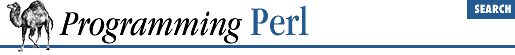#Chapter 3Functions### 3.2.91 map

```map `BLOCK` `LIST`
map `EXPR`, `LIST````

This function evaluates the `BLOCK` or `EXPR` for each element of `LIST` (locally setting \$_ to each element) and returns the list value composed of the results of each such evaluation. It evaluates `BLOCK` or `EXPR` in a list context, so each element of `LIST` may produce zero, one, or more elements in the returned value. These are all flattened into one list. For instance:

`@words = map { split ' ' } @lines;`

splits a list of lines into a list of words. Often, though, there is a one-to-one mapping between input values and output values:

`@chars = map chr, @nums;`

translates a list of numbers to the corresponding characters. And here's an example of a one-to-two mapping:

`%hash = map { genkey(\$_), \$_ } @array;`

which is just a funny functional way to write this:

```%hash = ();
foreach \$_ (@array) {
\$hash{genkey(\$_)} = \$_;
}```

See also grep. map differs from grep in that map returns a list consisting of the results of each successive evaluation of `EXPR`, whereas grep returns a list consisting of each value of `LIST` for which `EXPR` evaluates to true.